## (x sin a + y cos a)^2 + (x cos a – y sin a)^2 = x^2 + y^2

Question

(x sin a + y cos a)^2 + (x cos a – y sin a)^2 = x^2 + y^2

in progress 0
6 months 2021-08-14T05:07:23+00:00 1 Answers 3 views 0

## Answers ( )

Proved

Step-by-step explanation:

Required

Prove that: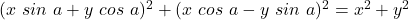Solving from left to right:

Open brackets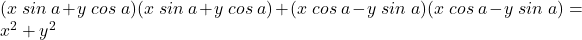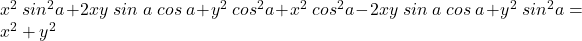Collect Like Terms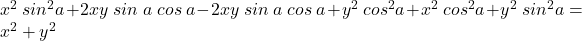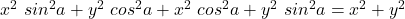Collect Like Terms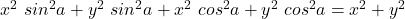Factorize: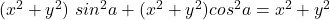Further factorize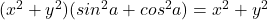In trigonometry: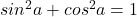So, we have: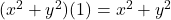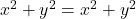Proved